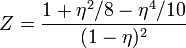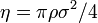# Solana hard disk equation of state$Z = \frac{1 + \eta^2/8 - \eta^4/10}{(1-\eta)^2}$
where the packing fraction is given by$\eta = \pi \rho \sigma^2 /4$ where$\sigma$ is the diameter of the disks.